﻿ Law Of Equivalence Of Energy And Mass, Man Writing On Transparent Screen Stock Image - Image of equivalence, dust: 99387285

# Law of Equivalence of Energy and Mass, Man writing on transparent screen.

Royalty-Free Stock Photo## Law of Equivalence of Energy and Mass, Man writing on transparent screen,high quality

More similar stock images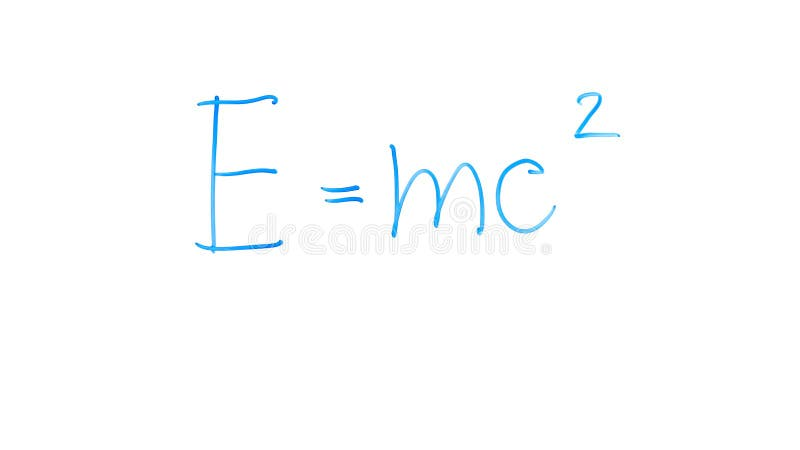Mass-energy equivalence formula written on glass, laws of classical physics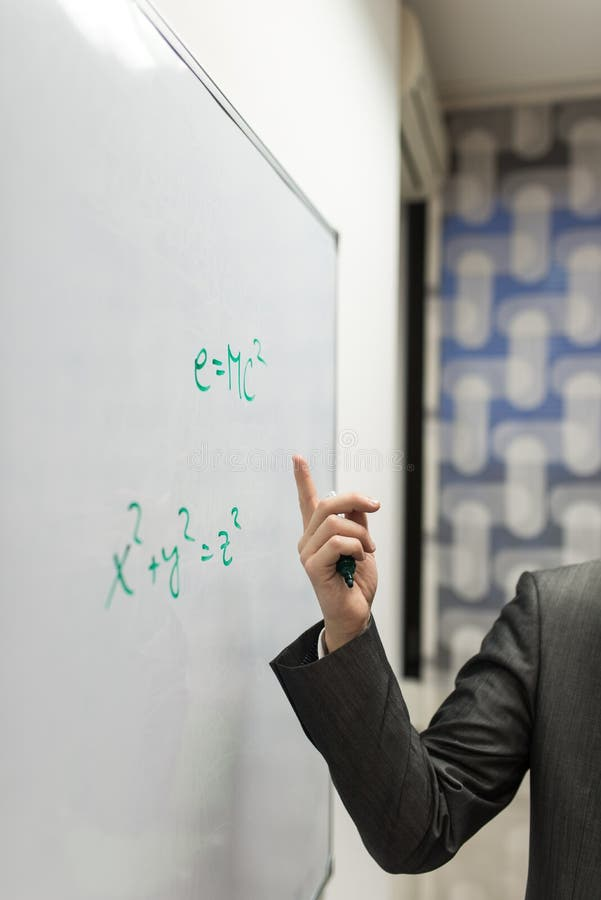Mass energy equivalence formulaMass energy equivalence physics formula written on tree wood trunk, hand drawn, doodle, autumn fall season, back to school concept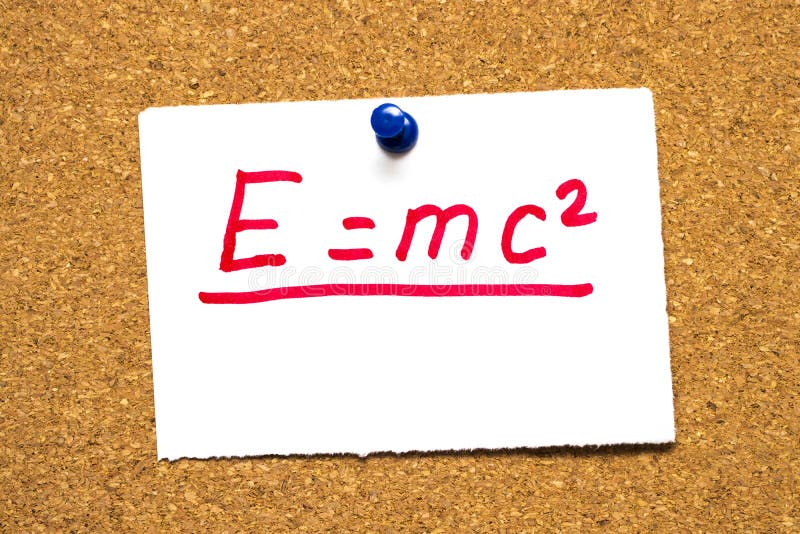E=mc2 Mass-energy equivalence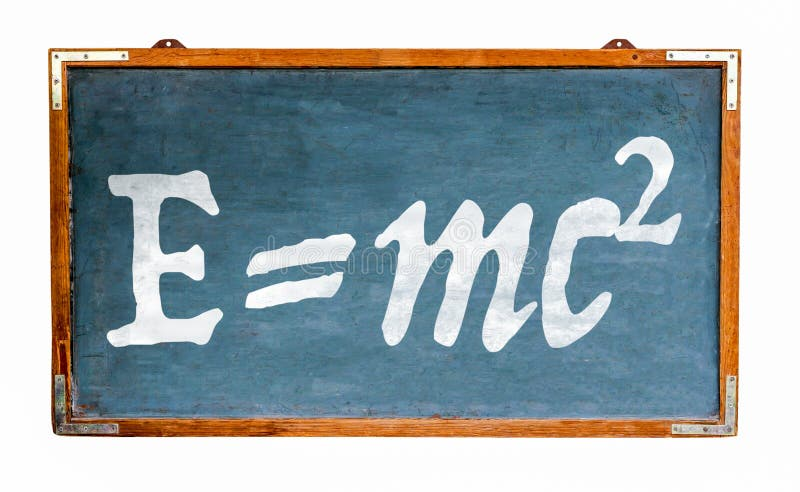Relativity theory E=mc2 equation mass energy equivalence on blue old grungy vintage wide wooden chalkboard retro blackboard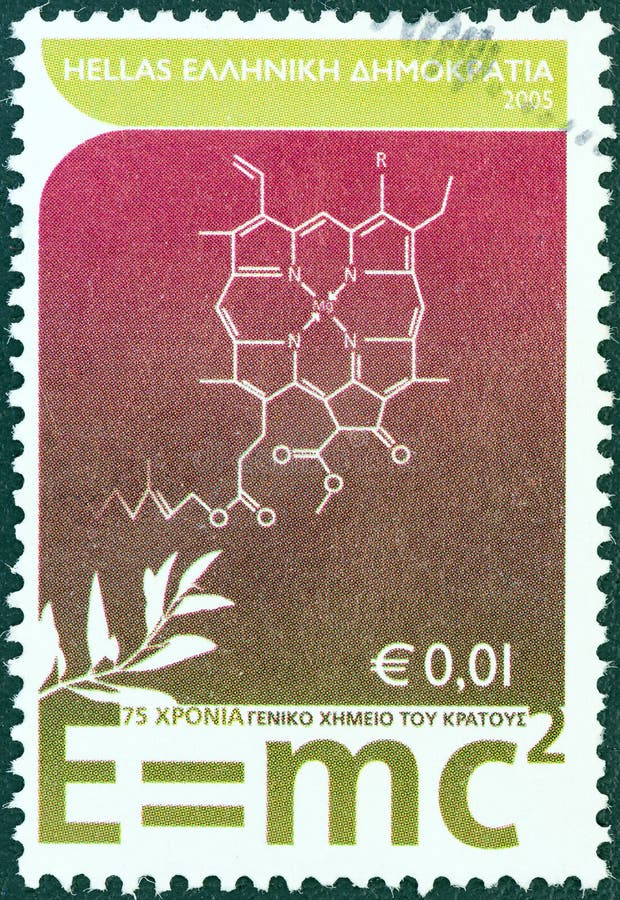GREECE - CIRCA 2005: A stamp printed in Greece shows `E = mc2` mass energy equivalence formula of Einstein, circa 2005.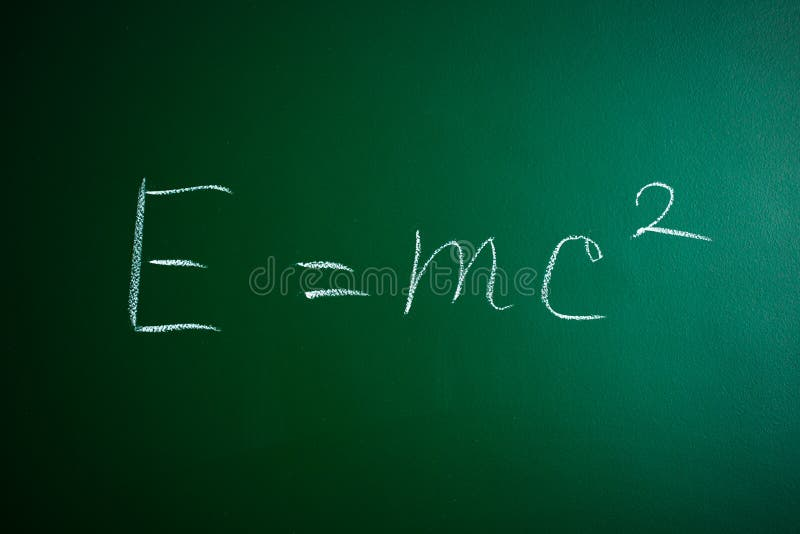Mass-energy equivalence formula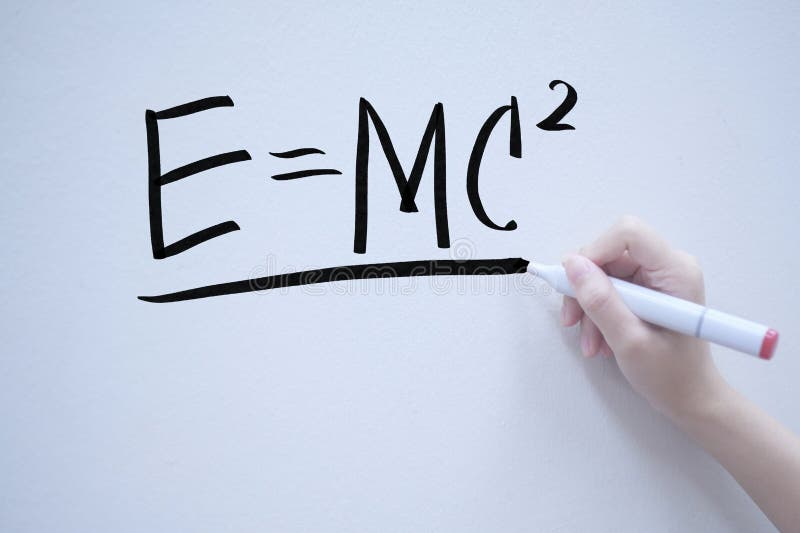mass-energy equivalent handwriting on whiteboardAlbert Einstein monument in Irrhausen, Germany